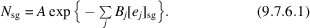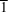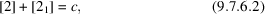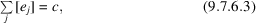International
Tables for
Crystallography
Volume C
Mathematical, physical and chemical tables
Edited by E. Prince

International Tables for Crystallography (2006). Vol. C, ch. 9.7, p. 904

## Section 9.7.6. A statistical model

A. J. C. Wilson,a V. L. Karenb and A. Mighellb

aSt John's College, Cambridge CB2 1TP, England, and bNIST Center for Neutron Research, National Institute of Standards and Technology, Gaithersburg, MD 20899, USA

### 9.7.6. A statistical model

| top | pdf |

Wilson (1988, 1990, 1991) has discussed the factors that, on statistical analysis, appear to govern the relative frequency of occurrence of the space groups of molecular organic crystals. In its developed form (Wilson, 1990), the statistical model postulated that, within an arithmetic crystal class (see Chapter 1.4), the number of examples, Nsg, of a space-group type would depend exponentially on the numbers of symmetry elements within the unit cell, thus:In this equation, A is a normalizing constant depending on the arithmetic crystal class,is the number of symmetry elements of typewithin the unit cell in the space group, andis a parameter depending on the arithmetic crystal class and the symmetry element; A andare independent of the space group. Empirically,has a positive sign for the syntropic symmetry elements (k and, where n = 2, 3, 4, 6) and a negative sign forand the antitropic symmetry elements (glide planes and screw axes). Often, however, laws of conservation of symmetry elements', of the typeor in generalwhere c is a constant for the crystal class, eliminate a separate dependence on one or more of the's (Wilson, 1990). Often a cohort larger than an arithmetic crystal class can be used, with arithmetic crystal class included as an additional factor.6 With this adjustment, the model can be used for such cohorts as geometric crystal class or even crystal system, giving fits within the usual crystallographic range (), but, for some classes (in particular mmm), statistical tests based on the scaled deviance indicated residual systematic error (Wilson, 1980). This was traced (Wilson, 1991) to the failure of an explicit postulate: The second possibility is that the distribution is seriously affected by molecular symmetry. Some molecules possess inherent symmetry, and this symmetry could coincide with the corresponding crystallographic symmetry element, again increasing the variance and/or bias of the number of examples per space group.The comparative rarity of utilization of molecular symmetry suggests that it can be ignored in an exploratory statistical survey' (abbreviated from Wilson, 1988). This procedure was perhaps reasonable in a first exploratory' survey, and in fact agreement in the usual crystallographic range () was achieved (Wilson, 1988, 1990). However, the scaled deviance indicated that systematic errors still remained (Wilson, 1980), and the discrepancy was traced to the use of molecular symmetry in many structures (Wilson, 1990, 1992). If such structures are eliminated, R2 falls to trivial values, and the agreement between observed and calculated frequencies becomes too good to be interesting.

### References

Wilson, A. J. C. (1980). Testing the hypothesis no remaining systematic error' in parameter determination. Acta Cryst. A36, 937–944.
Wilson, A. J. C. (1988). Space groups rare for organic structures. I. Triclinic, monoclinic and orthorhombic crystal classes. Acta Cryst. A44, 715–724.
Wilson, A. J. C. (1990). Space groups rare for organic structures. II. Analysis by arithmetic crystal class. Acta Cryst. A46, 742–754.
Wilson, A. J. C. (1991). Space groups rare for molecular organic structures: the arithmetic crystal class mmmP. Z. Kristallogr. 197, 85–88.
Wilson, A. J. C. (1992). International tables for crystallography, Vol. C, Mathematical, physical and chemical tables, edited by A. J. C. Wilson, Chap. 9.7. Dordrecht: Kluwer Academic Publishers.